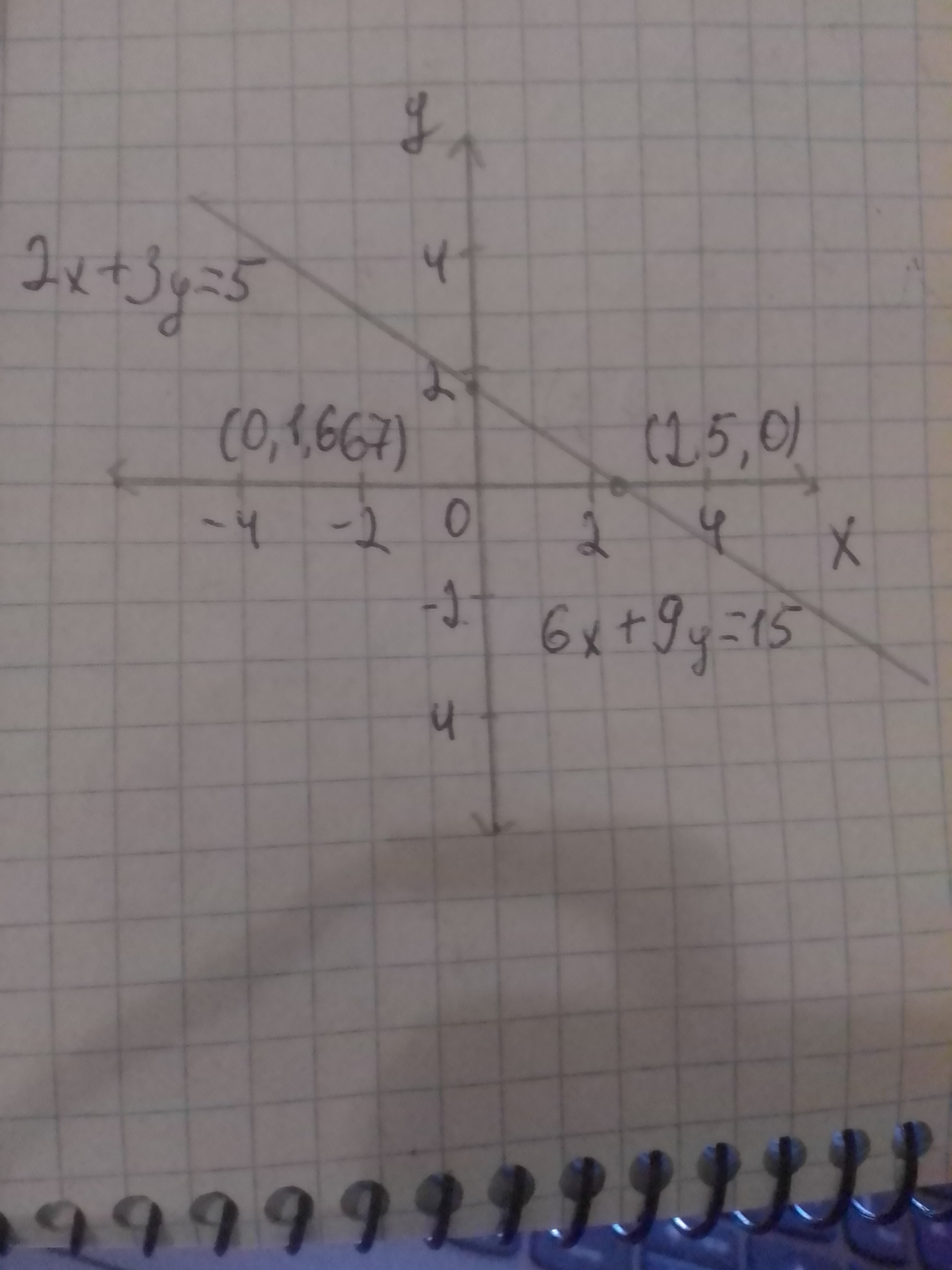# To solve:the system of equationdefazajx 2020-12-21 Answered

To solve:
the system of equation $2x+3y=5\phantom{\rule{1em}{0ex}}\text{and}\phantom{\rule{1em}{0ex}}6x+9y=15$.

You can still ask an expert for help

• Questions are typically answered in as fast as 30 minutes

Solve your problem for the price of one coffee

• Math expert for every subject
• Pay only if we can solve itnitruraviX

Calculation:
Plot
$2x+3y=5$
$0+3y=5$
$y=\frac{5}{3}$
$y=1.1667$
Put $y=0$
$2x+3y=5$
$2x+0=5$
$x=2.5$
Plot the above equations for coordinates (0, 1.667) and (2.5, 0) satisfying the equation.
Plot $6x+9y=15$
Put $x=0$
$6x+9y=15$
$0+9y=15$
$y=\frac{15}{9}$
$y=1.667$
Put $y=0$
$6x+9y=15$
$6x+0=15$
$6x=15$
$x=\frac{15}{6}$
$x=2.5$
Plot the above equation for coordinates (0, 1.667) and (2.5, 0) satisfying the equation.Since, graph is same for the equations therefore it has infinitely many solutions.
So, the answer is (j), infinite solutions.